Register
 Email: Passwd 验 证:换一张 Have you forgotten your password? Stay signed in Log In
 ALL Title Author Keyword Sponsors Type Publication date Submitted Time Subject Conference Name Source Categories KOS Subject Advisor ORCID Advanced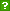ISCAS OpenIR  > 软件所图书馆  > 期刊论文
 Subject: Computer Science Title: multi-letter quantum finite automata: decidability of the equivalence and minimization of states Author: Qiu Daowen ; Li Lvzhou ; Zou Xiangfu ; Mateus Paulo ; Gruska Jozef Source: ACTA INFORMATICA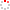Issued Date: 2011 Volume: 48, Issue:5-6, Pages:271-290 Indexed Type: SCI Department: Qiu Daowen; Li Lvzhou; Zou Xiangfu Sun Yat Sen Univ Dept Comp Sci Guangzhou 510006 Guangdong Peoples R China. Qiu Daowen; Mateus Paulo TULisbon Inst Super Tecn Dept Matemat SQIG Inst Telecomunicacoes P-1049001 Lisbon Portugal. Qiu Daowen Chinese Acad Sci Inst Software State Key Lab Comp Sci Beijing 100080 Peoples R China. Gruska Jozef Masaryk Univ Fac Informat Brno Czech Republic. Sponsorship: National Natural Science Foundation60873055, 61073054; Natural Science Foundation of Guangdong Province of China10251027501000004; Fundamental Research Funds for the Central Universities10lgzd12, 11lgpy36; Ministry of Education of China20100171110042, 20100171120051; China Postdoctoral Science Foundation20090460808, 201003375; SQIG at IT; FCT; EUQSec PTDC/EIA/67661/2006, AMDSC UTAustin/MAT/0057/2008; MSM0021622419 Abstract: Multi-letter quantum finite automata (QFAs) can be thought of quantum variants of the one-way multi-head finite automata (Hromkovic, Acta Informatica 19:377-384, 1983). It has been shown that this new one-way QFAs (multi-letter QFAs) can accept with no error some regular languages, for example (a + b)*b, that are not acceptable by QFAs of Moore and Crutchfield (Theor Comput Sci 237:275-306, 2000) as well as Kondacs and Watrous (66-75, 1997; Observe that 1-letter QFAs are exactly measure-once QFAs (MO-1QFAs) of Moore and Crutchfield (Theor Comput Sci 237: 275-306, 2000)). In this paper, we study the decidability of the equivalence and minimization problems of multi-letter QFAs. Three new results presented in this paper are the following ones: (1) Given a k(1)-letter QFA A(1) and a k(2)-letter QFA A(2) over the same input alphabet Sigma, they are equivalent if and only if they are (n(2)m(k-1) - m(k-1) + k)-equivalent, where m = vertical bar Sigma vertical bar is the cardinality of Sigma, k = max(k(1), k(2)), and n = n(1) + n(2), with n(1) and n(2) being numbers of states of A(1) and A(2), respectively. When k = 1, this result implies the decidability of equivalence of measure-once QFAs (Moore and Crutchfield in Theor Comput Sci 237:275-306, 2000). (It is worth mentioning that our technical method is essentially different from the previous ones used in the literature.) (2) A polynomial-time O(m(2k-1)n(8) + km(k)n(6)) algorithm is designed to determine the equivalence of any two multi-letter QFAs (see Theorems 2 and 3; Observe that if a brute force algorithm to determine equivalence would be used, as suggested by the decidability outcome of the point (1), the worst case time complexity would be exponential). Observe also that time complexity is expressed here in terms of the number of states of the multi-letter QFAs and k can be seen as a constant. (3) It is shown that the states minimization problem of multi-letter QFAs is solvable in EXPSPACE. This implies also that the state minimization problem of MO-1QFAs (see Moore and Crutchfield in Theor Comput Sci 237: 275-306, 2000, page 304, Problem 5), an open problem stated in that paper, is also solvable in EXPSPACE. English Abstract: Multi-letter quantum finite automata (QFAs) can be thought of quantum variants of the one-way multi-head finite automata (Hromkovic, Acta Informatica 19:377-384, 1983). It has been shown that this new one-way QFAs (multi-letter QFAs) can accept with no error some regular languages, for example (a + b)*b, that are not acceptable by QFAs of Moore and Crutchfield (Theor Comput Sci 237:275-306, 2000) as well as Kondacs and Watrous (66-75, 1997; Observe that 1-letter QFAs are exactly measure-once QFAs (MO-1QFAs) of Moore and Crutchfield (Theor Comput Sci 237: 275-306, 2000)). In this paper, we study the decidability of the equivalence and minimization problems of multi-letter QFAs. Three new results presented in this paper are the following ones: (1) Given a k(1)-letter QFA A(1) and a k(2)-letter QFA A(2) over the same input alphabet Sigma, they are equivalent if and only if they are (n(2)m(k-1) - m(k-1) + k)-equivalent, where m = vertical bar Sigma vertical bar is the cardinality of Sigma, k = max(k(1), k(2)), and n = n(1) + n(2), with n(1) and n(2) being numbers of states of A(1) and A(2), respectively. When k = 1, this result implies the decidability of equivalence of measure-once QFAs (Moore and Crutchfield in Theor Comput Sci 237:275-306, 2000). (It is worth mentioning that our technical method is essentially different from the previous ones used in the literature.) (2) A polynomial-time O(m(2k-1)n(8) + km(k)n(6)) algorithm is designed to determine the equivalence of any two multi-letter QFAs (see Theorems 2 and 3; Observe that if a brute force algorithm to determine equivalence would be used, as suggested by the decidability outcome of the point (1), the worst case time complexity would be exponential). Observe also that time complexity is expressed here in terms of the number of states of the multi-letter QFAs and k can be seen as a constant. (3) It is shown that the states minimization problem of multi-letter QFAs is solvable in EXPSPACE. This implies also that the state minimization problem of MO-1QFAs (see Moore and Crutchfield in Theor Comput Sci 237: 275-306, 2000, page 304, Problem 5), an open problem stated in that paper, is also solvable in EXPSPACE. Language: 英语 WOS ID: WOS:000297512700001 Citation statistics: Content Type: 期刊论文 URI: http://ir.iscas.ac.cn/handle/311060/16144 Appears in Collections: 软件所图书馆_期刊论文

 Files in This Item:

There are no files associated with this item.

 Recommended Citation: Qiu Daowen,Li Lvzhou,Zou Xiangfu,et al. multi-letter quantum finite automata: decidability of the equivalence and minimization of states[J]. ACTA INFORMATICA,2011-01-01,48(5-6):271-290.
 Service Recommend this item Sava as my favorate item Show this item's statistics Export Endnote File Google Scholar Similar articles in Google Scholar [Qiu Daowen]'s Articles [Li Lvzhou]'s Articles [Zou Xiangfu]'s Articles CSDL cross search Similar articles in CSDL Cross Search [Qiu Daowen]‘s Articles [Li Lvzhou]‘s Articles [Zou Xiangfu]‘s Articles Related Copyright Policies Null Social Bookmarking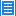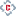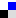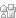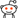您对该条目有什么异议，请填写以下表单，管理员会尽快联系您。 `内 容：` Email： * 单位： 验证码：刷新
 您在IR的使用过程中有什么好的想法或者建议可以反馈给我们。 `标 题：` * `内 容：` Email： * 验证码：刷新Copyright © 2007-2019  中国科学院软件研究所 - Feedback Powered by CSpace